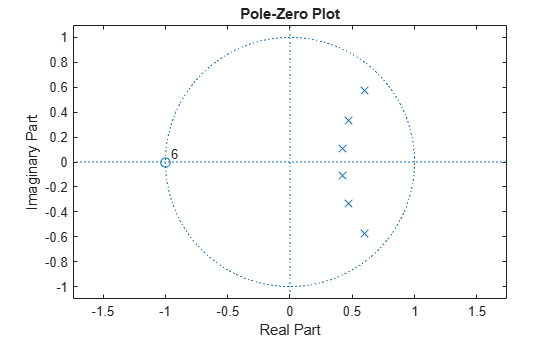# isminphase

Determine whether filter is minimum phase

## Syntax

```flag = isminphase(b,a) flag = isminphase(sos) flag = isminphase(d) flag = isminphase(...,tol) flag = isminphase(hs,...) isminphase(hs,'Arithmetic',arithtype) ```

## Description

A filter is minimum phase when all the zeros of its transfer function are on or inside the unit circle, or the numerator is a scalar. An equivalent definition for a minimum phase filter is a causal and stable system with a causal and stable inverse.

`flag = isminphase(b,a)` returns a logical output, `flag`, equal to `true` if the filter specified by numerator coefficients, `b`, and denominator coefficients, `a`, is a minimum phase filter.

`flag = isminphase(sos)` returns `true` if the filter specified by second order sections matrix, `sos`, is minimum phase. `sos` is a K-by-6 matrix, where the number of sections, K, must be greater than or equal to 2. Each row of `sos` corresponds to the coefficients of a second order (biquad) filter. The ith row of the `sos` matrix corresponds to ```[bi(1) bi(2) bi(3) ai(1) ai(2) ai(3)]```.

`flag = isminphase(d)` returns `true` if the digital filter, `d`, has minimum phase. Use `designfilt` to generate `d` based on frequency-response specifications.

`flag = isminphase(...,tol)` uses the tolerance, `tol`, to determine when two numbers are close enough to be considered equal. If not specified, `tol`, defaults to `eps^(2/3)`.

```flag = isminphase(hs,...)``` determines whether the filter System object™ `hs` is minimum phase, returning `1` if true and `0` if false. You must have the DSP System Toolbox™ software to use this syntax.

`isminphase(hs,'Arithmetic',arithtype)` analyzes the filter System object `hs` based on the specified `arithtype`. `arithtype` can be `'double'`, `'single'`, or `'fixed'`. When you specify `'double'` or `'single'`, the function performs double- or single-precision analysis. When you specify `'fixed'` , the arithmetic changes depending on the setting of the `CoefficientDataType` property and whether the System object is locked or unlocked. You must have the DSP System Toolbox software to use this syntax.

When you do not specify the arithmetic for non-CIC structures, the function uses double-precision arithmetic if the filter System object is in an unlocked state. If the System object is locked, the function performs analysis based on the locked input data type. CIC structures only support fixed-point arithmetic.

## Examples

collapse all

Design a sixth-order lowpass Butterworth IIR filter using second order sections. Specify a normalized 3-dB frequency of 0.15. Check if the filter has minimum phase.

```[z,p,k] = butter(6,0.15); SOS = zp2sos(z,p,k); min_flag = isminphase(SOS)```
```min_flag = logical 1 ```

Redesign the filter using `designfilt`. Check that the zeros and poles of the transfer function are on or within the unit circle.

```d = designfilt('lowpassiir','DesignMethod','butter','FilterOrder',6, ... 'HalfPowerFrequency',0.25); d_flag = isminphase(d)```
```d_flag = logical 1 ```
`zplane(d)`Given a filter defined with a set of single-precision numerator and denominator coefficients, check if it has minimum phase for different tolerance values.

```b = single([1 1.00001]); a = single([1 0.45]); min_flag1 = isminphase(b,a) ```
```min_flag1 = logical 0 ```
`min_flag2 = isminphase(b,a,1e-3)`
```min_flag2 = logical 1 ```